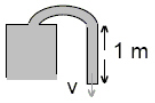/
/
/
66. A Venturi tube may be used as the inlet
Not my Question
Flag Content

# Question :   66. A Venturi tube may be used as the inlet : 2102180

66. A Venturi tube may be used as the inlet to an automobile carburetor. If the inlet pipe of 2.0 cm diameter narrows to 1.0 cm diameter, what is the pressure drop in the constricted section for airflow of 3.0 m/s in the 2-cm section? (Assume air density is 1.25 kg/m3.)

A. 70 Pa

B. 84 Pa

C. 100 Pa

D. 115 Pa

67. Water is sent from a fire hose at 30 m/s at an angle of 30? above the horizontal. What is the maximum height reached by the water?

A. 7.5 m

B. 11 m

C. 15 m

D. 19 m

68. How much power is theoretically available from a mass flow of 1 000 kg/s of water that falls a vertical distance of 100 m?

A. 980 kW

B. 98 kW

C. 4 900 W

D. 980 W

69. A fluid is drawn up through a tube as shown below. The atmospheric pressure is the same at both ends. Use Bernoulli's equation to determine the speed of fluid flow out of the tank. If the height difference from the top of the tank to the bottom of the siphon is 1.0 m, then the speed of outflow is:A. 1.1 m/s

B. 2.2 m/s

C. 4.4 m/s

D. 8.8 m/s

70. It takes 2.0 minutes to fill a gas tank with 40 liters of gasoline. If the pump nozzle is 1.0 cm in radius, what is the average speed of the gasoline as it leaves the nozzle? (1 000 liters = one cubic meter)

A. 0.27 m/s

B. 1.1 m/s

C. 11 m/s

D. 64 m/s

## Solution 5 (1 Ratings )

Solved
Physics 1 Year Ago 315 Views